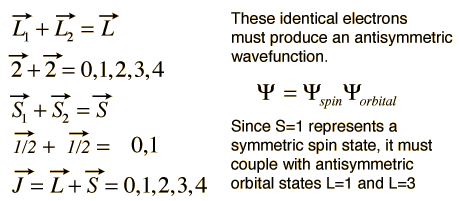# Russell-Saunders or L-S Coupling

For multi-electron atoms where the spin-orbit coupling is weak, it can be presumed that the orbital angular momenta of the individual electrons add to form a resultant orbital angular momentum L. Likewise, the individual spin angular momenta are presumed to couple to produce a resultant spin angular momentum S. Then L and S combine to form the total angular momentum.

J = L + S

This kind of combination is visualized in terms of a vector model of angular momentum.This kind of coupling is called L-S coupling or Russell-Saunders coupling, and it is found to give good agreement with the observed spectral details for many light atoms. For heavier atoms, another coupling scheme called "j-j coupling" provides better agreement with experiment.

The vector model illustrated above was applied to a single electron, but a similar model can be applied when multiple electrons contribute to the net angular momentum. If identical electrons are involved, then proper care must be taken to make sure the combinations produce an antisymmetric wavefunction upon exchange of identical electrons. For example, consider the electron configuration of titanium, (Ar)3d24s2. The 4s electrons contribute to a closed shell and the 3d2 electrons contribute the net angular momentum. Under the L-S coupling conditions this gives the following.The possibility of J=5 is excluded by symmetry considerations for the identical electrons, but the other states can be visualized by the vector model.

Index

Atomic structure concepts

 HyperPhysics***** Quantum Physics R Nave
Go Back

# j-j Coupling

In light atoms, the interactions between the orbital angular momenta of individual electrons is stronger than the spin-orbit coupling between the spin and orbital angular momenta. These cases are described by "L-S coupling". However, for heavier elements with larger nuclear charge, the spin-orbit interactions become as strong as the interactions between individual spins or orbital angular momenta. In those cases the spin and orbital angular momenta of individual electrons tend to couple to form individual electron angular momenta.Another case where the overall L and S are decoupled is the case where there is a very strong external magnetic field applied. This is called the Paschen-Back effect.

Index

Atomic structure concepts

Reference
Rohlf
Ch 9

 HyperPhysics***** Quantum Physics R Nave
Go Back